# Binary Search Tree

Sept. 14, 2018 23866

### Previous

A binary search tree is a binary tree where for every node, the values in its left subtree are smaller than every value in its right subtree.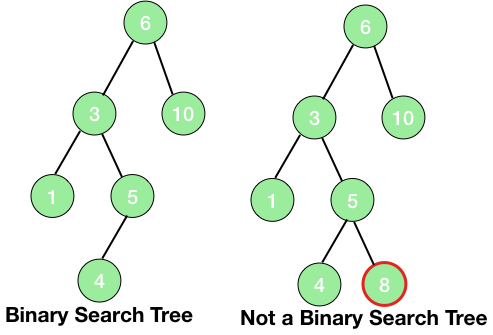In the above picture, the second tree is not a binary search tree. All the values in the left subtree of any node must be smaller than the values of the right subtree of that node but the here the value 8 is not smaller than 6. Hence, the second tree is not a binary search tree.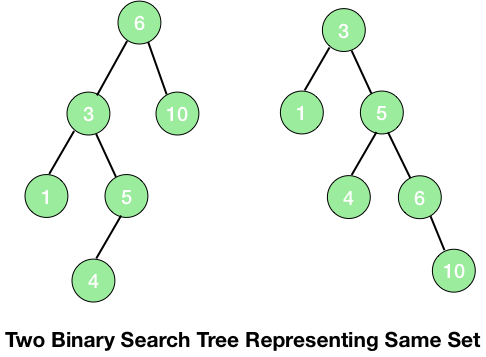## Searching in a Binary Tree

Let's suppose we have to find a value in a binary search tree. If we are on any node of a binary search tree and the value to be found is greater than the value at the node then we are assured that the value will lie somewhere on the right subtree and if it is smaller then on the left subtree and thus making the searching in a tree much efficient.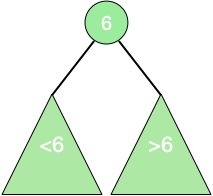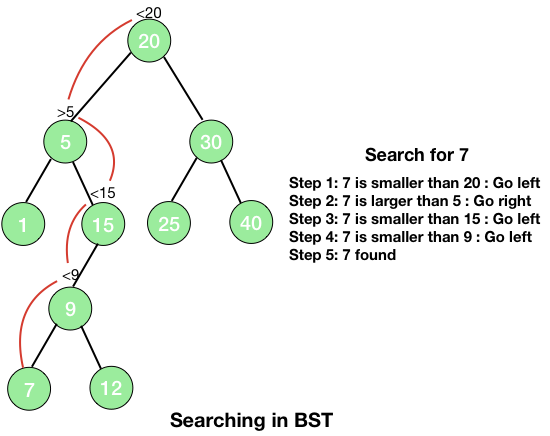One interesting point should be noted here that when the inorder traversal is applied on a binary search tree, it prints all the data of the binary tree in the sorted order.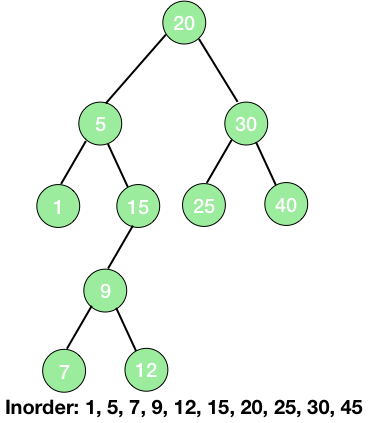Thus, the pseudo code for searching in a binary tree can be written as:

Node search(int x, Node n)
{
if(n != null)
{
if(n.data  == x)
return (n)
else if(n.data > x)
search(x, n.left)
else
search(x, n.right)
}
}


if(n.data  == x) – We are simply comparing the data at the current node with the value to be found and if both are equal then we have found the value and thus returning the current node (which contains the value) in the next line (return (n)).

else if(n.data > x) – If the data to be found is smaller than the data at the current node, then it must lie at the left subtree and thus we are again calling the search funtion on the left subtree.

else – the data to be found is greater than the data at the current node and thus it must lie in the right subtree. So, we are implementing the search funtion to the right subtree in the next line.

## Finding Minimum/Maximum in a Binary Tree

In a binary tree, we can strongly say that the smallest element will be the leftmost leaf of the tree i.e., to find the smallest element, we will start from the root and will go left as long as there is a left child. Thus, the code for the same can be written as:

int findMin(Node n)
{
if(n.left==null)
return (n.data)
else
findMin(n.left)
}


if(n.left==null) – There is no left child of the node and thus the current node is the leftmost node and thus it must hold the smallest value of the tree.

else – Move to the left child.

Similary, we can find the maximum element of a binary search tree at its rightmost node.

int findMax(Node n)
{
if(n.right==null)
return (n.data)
else
findMax(n.right)
}


## Inserting an Element in a Binary Search Tree

A new element should be inserted at a particular position in a binary search tree such that by inserting the new node the binary search tree remains a binary search tree. This can be achieved by simply making a search for the element to be inserted and if we didn't find the element, then insert a new node at the correct position. Thus, the steps for inserting an element x in a binary search tree are:

1. Search for the element x.
2. If the element is found, do nothing.
3. Else, insert x at the last spot on the path traversal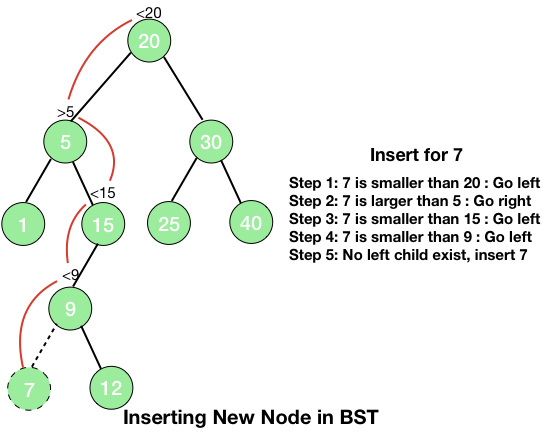insert (Node n, int x)
{
if (n==null)
{
n = new Node(x)
}
if(n.data < x)
insert(n.right, x)
else if(n.data > x)
insert(n.left, x)
}


## Deleting a Node in BST

The deletion part is also easy but most complex among the above-mentioned tasks. When we delete a node, we have to take care of the children of the node and also that the property of a BST is maintained. There can be three cases in deletion of a node which are explained below:

1. The node is a leaf - This is the most simple case and here we can delete the node immediately without giving a second thought.

2. The node has one child - This is represented in the picture given below.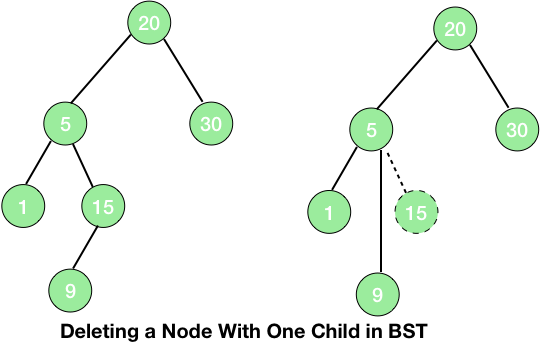When the node to be deleted has only one child then just replacing the node with its child will maintain the property of the search tree. So, we just replace the node with its child i.e., link the parent of the node to be deleted to its child. Since both the node to be deleted and its child are on the same side and there are no further children, so the property of a search tree will be maintained.
3. The node has 2 children - When the node to be deleted has 2 children, we need to choose a node to be replaced with the node to be deleted such that the property of the binary tree remains intact. When you look at the tree, you will find that either choosing the maximum element of the left subtree or the minimum element of the right subtree will satisfy this condition. We will proceed with choosing the minimum element of the right subtree and then we will delete the node. Now, the smallest element on the right subtree must have a single child or no child at all so, we can delete this node using either the first or the second case.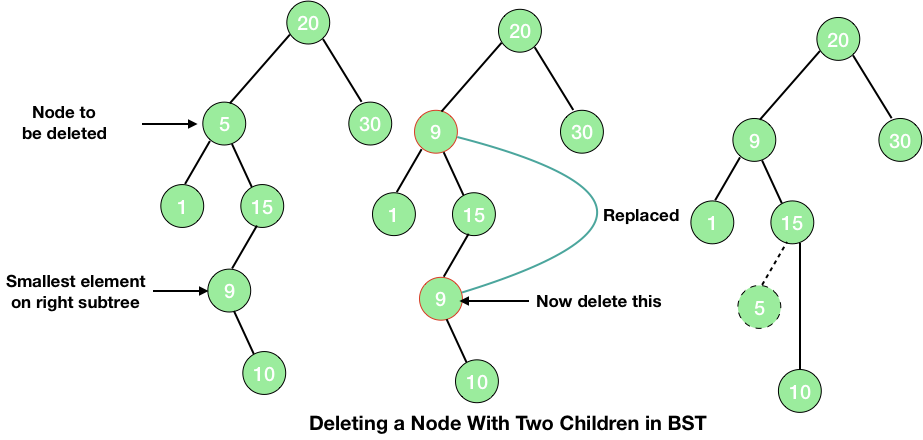code

Now you know the concepts of the binary search tree, the implementations in C and Java are can be found in the posts: Binary Search Tree in Java and Binary Search Tree in C.

### Next:

Liked the post?
Developer and founder of CodesDope.
0 COMMENT